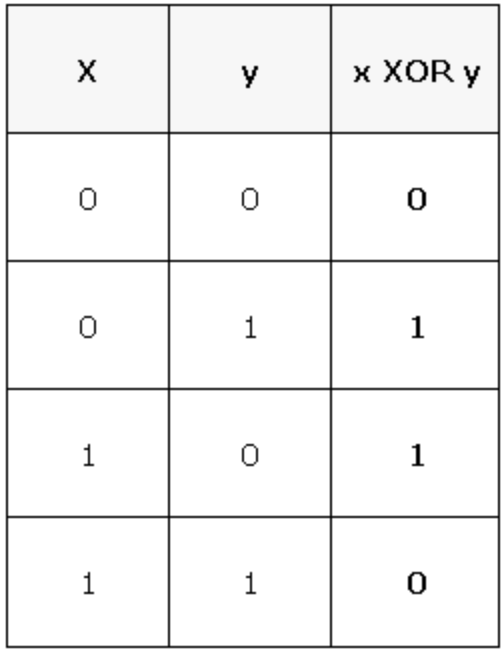×

Search anything:

# Bitwise Algorithm to Find the Number Occurring with Odd Frequency

#### bitwise operation xor operation Algorithms bitwise algorithmIn this post I am going to "explore the bitwise algorithm to find the only number occuring odd number of times in a given set of numbers". I am handling the task using the bitwise operator `XOR` and I have chosen to implement the algorithm in Python3 since I am quite comfortable with the language.

A bitwise operator operates on binary numbers and considers one bit at a time. There are many bitwise operators like the `AND`, `OR`, `NOT`, `NOR` operators but for the given problem, I am considering the `XOR` or the `Exclusive OR` operator.
The `XOR` operator gives an output 0 when a number is operated with itself (e.g. `5 XOR 5 = 0`). Moreover, when a number is operated with 0, the operator gives the number itself as the output (e.g. `5 XOR 0 = 5`). Furthermore, the `XOR` operator is commutative (i.e. `x XOR y = y XOR x`) and associative (i.e. `x XOR (y XOR z) = (x XOR y) XOR z`). The truth table for an `XOR` gate is as shown below.The basic idea is that if one initialises a variable as 0 and applies the `XOR` operator to the variable with each element of an array of numbers, at the end the variable will store the number which has been repeated odd number of times. This is simply because an even frequency will nullify the value stored in the variable. The process will be clearer with the aid of the pseudocode and hand simulation.

### Pseudocode

Pseudocode:

``````Considering an array of numbers, "array", and each element of the array, "element";
1. Set result = 0
2. Repeat step 3 while element is in array
3. Set result = result XOR element
4. Return result
5. Exit
``````

### Hand Simulation

Considering the array = [2, 3, 2, 1, 3, 2, 1];

Clearly, intuition suggests that the number repeated odd number of times is 2. Let us check if the above pseudocode works or not.

• According to step 1, we set `result` = 0.
• Since `element` is in `array`, we enter into the loop. In the following table I compare the values of the variable `result` at every pass of the loop:
Pass Element in the Array 'result'
0 - 0 = 0000
1 2 0 `XOR` 2 = 0000 `XOR` 0010 = 0010 = 2
2 3 2 `XOR` 3 = 0010 `XOR` 0011 = 0001 = 1
3 2 1 `XOR` 2 = 0001 `XOR` 0010 = 0011 = 3
4 1 3 `XOR` 1 = 0011 `XOR` 0001 = 0010 = 2
5 3 2 `XOR` 3 = 0010 `XOR` 0011 = 0001 = 1
6 2 1 `XOR` 2 = 0001 `XOR` 0010 = 0011 = 3
7 1 3 `XOR` 1 = 0011 `XOR` 0001 = 0010 = 2
• According to step 4, `result` returns the value 2.
The final value stored in the variable `result` is 2 which matches with the intuition.

### Complexity

• Time complexity: `Θ(n)`
• Space complexity: `Θ(1)`

### Python3

``````
array = [2, 3, 2, 1, 3, 2, 1]
result = 0
for element in array:
result = result ^ element
print(result)
``````